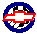ATTENTION: These pages were created & copyrighted by Steve Parker. They reside here on MonteCarloSS.com only until we can contact Steve to get his site back up in operation.

#Useful Formulas

• Conversion 1 Liter = 61.02 Cubic Inches
• HorsePower = TORQUE * RPM / 5252
• HorsePower from MPH (1/4 mi.) = (.00426 * MPH)^3 * WEIGHT
• HorsePower from MPH (1/4 mi.) = WEIGHT * (MPH/234)^3
• HorsePower from ET (1/4 mi.) = WEIGHT * (5.825/ET)^3
• ET from HorsePower (1/4 mi.) = (WEIGHT / HP)^.333 * 5.825
• TORQUE = (5252 * HP) / RPM
• CFM = (CID/2)*(RPM/1728)*VE
```VE = volumetric efficiency
VE is approximately equal to 0.78 for stock engines, 0.85 for hi-perf street engine
and approx. equal to 0.97+ for full race engines.
This gives rather low values for CFM but is probably all you really need.
```
• Tire height (inches) = {[(section width * profile) / 2540] + (wheel diameter / 2)} * 2
• 60 foot time from ET = (.12 * 1/4 mile et) + .17
• MPH = Tire Radius / 168 * RPM / Trans Gear * Rear Gear
• RPM = 168 * Trans Gear * Rear Gear * MPH / Tire Radius
• GEAR RATIO = Tire Radius * RPM / 168 / MPH
• Tire Radius = 168 * MPH * Trans Gear * Rear Gear / RPM
• CID = Number Of Cylinders * Bore * Bore * Stroke
• Volumetric Efficiency = Actual Engine Air Intake (CFM) / CID
• Compression Ratio = Cyl. Volume @ BDC / Cyl. Volume @ TDC = 1 + (Swept Volume / Volume @ TDC) = 1 + (0.7854 * Bore * Bore * Stroke) / (CCV + HGV / PDV)

CCV = Combustion Chamber Volume (in inches) = CC's / 16.4 HGV = Head Gasket Volume (in inches) = Compressed thickness * 0.7854 * Bore * Bore PDV = Piston Deck Volume = Volume of Piston Dish + Volume of Piston Dome

• HorsePower = Atmos. Press. * CR * VE * CID * RPM / 5252 / 150.8

 Stock SS File GM Vehicle Codes Engine Tech Small Block Info Drivetrain Tech Suspension Tech Tires & Wheels Emissions Legal SBC Street/Strip SBC Useful Formulas How To's Engine Swaps Bracket Racing Big Blocks